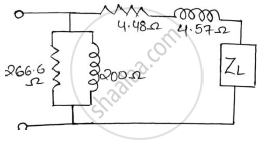# The Oc and Sc Test Data Are Given Below for a Single Phase, 5kva, 200v/400v, 50hz Transformer - Basic Electrical and Electronics Engineering

Sum

The OC and SC test data are given below for a single phase, 5KVA, 200V/400V, 50Hz transformer

 OC test from LV side 200V 1.25A 150w SC test from HV side 20V 12.5A 175w

Determine the following :-
1) Draw the equivalent circuit of the transformer referred to LV side.
2) At what load or KVA the transformer is to be operated for maximum efficiency?
3) Calculate the value of maximum efficiency.
4) Regulation of the transformer at full load 0.8power factor lagging.

#### Solution

(1) Approximate equivalent circuit.
From OC test (meters are connected on LV side i.e. secondary)

cosvarphi_0^'=W_0/(V_0I_0^')=150/(200xx1.25)=0.6     sinvarphi_0^'

I_W^'=I_0^'cosvarphi_0^'=1.25xx0.6=0.75A      R_0^'=V_0/I_W^'=200/0.75=266.6Ω

I_mu^'=I_0^'sinvarphi_0^'=1.25×0.8=1A  X_0^'=V_0/I_mu^'=200/1=200Ω

From SC test (meters are connected on the HV side i.e. primary)
𝑊𝑆𝐶=175𝑤 𝑉𝑆𝐶=20𝑉 𝐼𝑆𝐶=12.5𝐴

Z(01)=V_(SC)/I_(SC)=20/12.5=1.6Omega    R(01)=W_(SC)/I_(SC)^2=175/12.5^2=1.12Omega

X_(01)=sqrt(Z_(01)^2-R_(01)^2)=sqrt(1.6^2-1.12^2)=1.1426Omega

K=400/200=2

R_(02)=K^2R_(01)=2^2xx1.12=4.48Omega

X_(02)=K^2R_(01)=2^2xx1.1426=4.57Omega(2) Maximum efficiency and load at which it occurs.

𝑊𝐶𝑈= 𝐼22𝑅02

I_2=(5xx1000)/400-12.5

W_(CU)=12.5^2xx4.48=700

Load KVA = Full-load KVA×sqrt(W_1/W_(cu))=5xxsqrt(150/700)=2.314KVA

FOR MAXIMUM EFFICIENCY:-
𝑊𝑖=𝑊𝑐𝑢=150𝑊=0.150𝐾𝑊
Pf =1

%eta_(max)=("load KVA" xx "pf")/("load KVA" xx "pf"+W_i+W_i)xx100

%eta_(max)=(5xx1)/(5xx1+0.15+0.15)xx100

%eta_(max)=94.33%

(3) Regulation of transform at full load 0.8power factor lag.

% "regulation"=(I_2(R_(02)cosvarphi+X_(02)sinvarphi))/E_2xx100

% "regulation"=(12.5(4.48xx0.8+4.57xx0.6))/E_2xx100=19.76%

% "regulation"=19.76%

Concept: OC and SC Test
Is there an error in this question or solution?
2018-2019 (December) CBCGS

Share